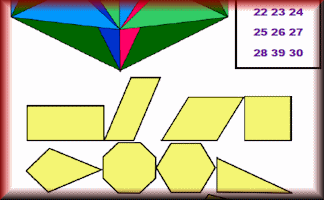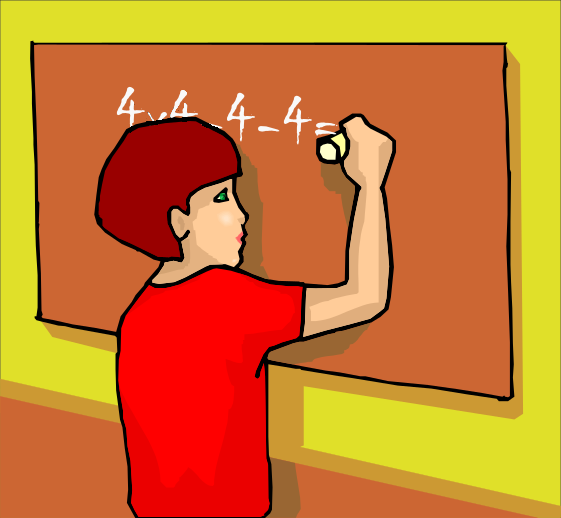# Emergency Maths Lessons

## Here are some mathematics lesson plans to be used on the rare occasions when a class is left without their normal teacher and you are stepping in at a moment's notice.

### Transum > Emergency Lessons > Negative Numbers

• Starter: Negative Vibes - Practise techniques for answering questions involving negative numbers.
• Number Line  Using a number line for adding and subtracting negative numbers is a must. Begin with a whole class explanation of how this works using this interactive visual aid. The number patterns in the Starter might help pupils devise rules for multiplying and dividing negative numbers but a summary can be found here: Mix and Math Level 2
• Temperatures  The first exercise pupils might attempt is one involving temperatures. Here they can see negative numbers in real life situations. The temperature analogy will be useful with further understanding of negative numbers.
• Negative Numbers  Now a more traditional exercise pupils can do on their own online devices (computers, laptops, iPads etc.). This exercise provides practice using negative numbers in basic arithmetic and algebraic calculations.
• Stop the lesson for a quiz question with a prize for the first correct answer (A protractor or set square would be a suitable prize). "Can you tell me what the temperature has been at noon for the past five days?" John asked the weatherman. "I don't exactly recall," replied the weatherman, "but I do remember that the temperature was different each day, and that the product of the temperatures is 12." Assuming that the temperatures are expressed to the nearest degree, what were the five temperatures? (From Amazing Brain Teasers (Puzzle Emporium)by Erwin Brecher) [One possible answer is 3,2,1,-1,-2 and it is the understanding of negative factors that is required]
• Negative Magic  A challenge to complete magic squares containing negative numbers.
• Bidmaze  The final negative number activity is challenge to get through a maze by completing BIDMAS calculations with some negative numbers in all but the easiest levels.
• Finisher: Manifest - Everyone (including the teacher) writes down (secretly) a single digit number, a two digit number, a three digit number and a four digit number but, for all of these four numbers, the digits 0 to 9 can only be used once. When everyone has done this the teacher reveals their four numbers by writing them on the board. Pupils then score their own numbers. If their single digit number is larger than the teacher's they score one point, if their two digit number is larger than the teacher's they score two further points and so on. Finally the pupil(s) with the highest scores take over the role of the teacher for the next round.
Manifest

It doesn’t happen very often but sometimes you find yourself in the position of having to deliver a lesson at a moment’s notice. In the worst case scenario you do not know what the class have previously been learning and you have been given no information about the nature of the pupils from their normal teacher.

These lesson plans have a low threshold, high ceiling so can be great learning opportunities for a wide range of pupils and class dynamics. No preparation is required as all of the activity details can be found by clicking on the links in the plans.

If pupils complete the activities online and earn trophies as a record of their progress teachers (who are Transum subscribers) can see from their class list which pupils have earned which trophies.

Furthermore if pupils do have access to internet connected computers see Laptops In Lessons for more lesson plans. If they have iPads there are some ideas on the iPad Maths page.

Show all Emergency Lesson Plans.

There is a printable page called Lectio Tumultuarios (Latin for Emergency Lesson) designed for Maths tutors (who are Transum subscribers) to have printed and stored in their folder ready to use on the odd occasion when a quick five minute time filler is required.Transum,

Friday, March 23, 2018

"My extreme emergency lesson (back in the day) was the Four Fours challenge. It was sort of an HCF of all the classes I was likely to have to cover and required no equipment or preparation.The objective of this challenge is to find the simplest mathematical expression for every integer from one to some maximum (depending on the class), using only common mathematical symbols and four copies of the digit four.

Nowerdays if you want to issue this challenge to a class you could do it using a different digit by using the 4th July Starter page as a projectable visual aid."

Do you have any comments? It is always useful to receive feedback and helps make this free resource even more useful for those learning Mathematics anywhere in the world. Click here to enter your comments.For All: